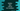# Python Program to find the LCM of two numbers## Find the LCM of two numbers in python :

What is an LCM value of two numbers?

LCM or least common multiplier of two numbers is the smallest number that is divisible by both of these numbers. i.e. the lowest number starting from 1, that is divisible by both.

To find out the LCM of two numbers in python or in any programming language, we can check for each number if it is divisible by both or not. Or, we can start this counting from the greater number, which will save us a lot of time. Or, we can only check for the multiplier of the greater number instead. Which method will be the fastest? Of course the third one!

In this tutorial, we will learn how to find out the LCM of two numbers in Python.

The algorithm of the program looks like below :

## Algorithm :

1. Store the numbers in two constant variables. If you want, you can also read these numbers as input from the user.
2. Find out the larger number between these two numbers.
3. Assign the larger number as the LCM of these two numbers.
4. Run one loop to find out the LCM of these numbers. This loop will run from the current value of LCM (or the larger number) to the multiplication of both numbers.

Note that this loop will not check all numbers in the range. It will only check the numbers that are divisible by the larger number.

e.g. if we are finding the LCM of 3 and 4, 4 will be considered as the initial value of the required LCM. The loop will then check the numbers within 4 and 4 * 3 = 12. It will check 4, 8 and 12. Since 4 and 8 don’t satisfy the condition, 12 is the required LCM.

Let’s take a look into the python program :

## Python Program :

``````def findLcm(a,b):
large_no = 0

if(a>b):
large_no = a
else :
large_no = b

multiplier = 1
lcm = large_no

while(lcm < (a*b)):
print ("checking for ",lcm)
if(lcm % a == 0 and lcm % b ==0):
break

multiplier += 1
lcm = large_no * multiplier

print ("lcm is ",lcm)

num1 = 31
num2 = 15

findLcm(num1,num2)``````

### Description :

1. To get the lcm of two numbers, we need to find the multiplier for both of the numbers. And the lowest multiplier will be the LCM. If one number is divisible by the other number, then the greater number will be the LCM.

In the above example, we have one method named ‘findLcm’ that takes two numbers as input and print out the LCM for both.

1. First, we are checking between these two number which one is greater and saving it to a variable ‘greaternum’_
2. Consider the greater number as lcm. If it is divisible by the smaller number, then it will be the lcm for both.
3. Now, inside the while loop, we are checking if the ‘lcm’ is divisible by both the numbers or not. If yes, then print it as the lcm, if not , then change ‘lcm’ to the next multiplier of the greater number. i.e. we are checking for all the multiplier of the greater number.
4. This loop will exit if ‘lcm’ becomes equal to the multiplication of both the numbers.

Try this example with different numbers and let me know if you find any trouble with it. You can also modify the program to read the numbers as input from the user.